# How Gravity Would Be Different If the World Were a CubePerhaps the main difference if the earth were a cube would be that students would become much more frustrated trying to calculate the gravitational field. For a uniform cube with side length L and density rho, the gravitational force on mass m at position (x,y,z) is given bywithwhere alpha, beta, and gamma are +/-1. This is the x-component of the force. By symmetry, the y and z components are given by swapping y for x and z for x in the above equation. (It’s just a matter of integrating Newton’s law of gravitation over the cube, but it gets a little messy.)

If we want a cubical earth, with the same mass as spherical earth, we get L = 9340 km. Let’s set aside the concern that such a planet might not be stable for very long, and take a look at its properties.

The graphs below show lines of force in blue, and equipotential lines in purple for two different slices through the cube, as shown. The black square (rectangle) indicates the boundary of the cube.First, notice that at the surface of the cube, the lines of force are not in general perpendicular to the faces of the cube. If you were standing on the cube, you would therefore perceive that you were standing on a slope. The apparent angle of this slope is given by the angle of the gravitational force relative to the face of the cube. The graph below shows this perceived angle as a function of position over one face of the cube.The apparent slope is higher near the corners of the cube, all the way up to about 50 degrees from the horizontal. Note that if you were standing on a face of the cube, you would not see the surface as shown in the figure above. The surface would appear completely flat, because it is. If you were accustomed to living on a spherical earth (as we are), I believe you would feel like the entire face of the cube you were standing on were sloped at a fixed angle, and this angle would depend on where on the face you were standing.

Second, we can take a look at the equipotential lines on the graphs above. These lines, shown in purple, are lines along which the gravitational potential energy is constant. If the earth’s water were divided equally among the faces of the cube, it would lie with its surface along an equipotential line. If we take the total volume of the water on earth (~1.4e9 km^3), I estimate that the six oceans would sit on each face, extending about L/5 from the center of the face, and having a height in the center of about 0.03L. This corresponds, perhaps, to the equipotential line closest to the face of the cube in the graphs above. That is, each ocean would be a lens shape with a round, somewhat squared off, perimeter. These lens shapes would be roughly 4000km across, and at the center, would extend about 300km up from the face of the cube.

The equipotential lines also indicate a line along which atmospheric pressure would be constant. From the right graph above, we can see that the atmospheric pressure at one of the corners is the same as the pressure about 0.22L (2200 km) above the center of the face. Seeing as how on spherical earth, the boundary between the atmosphere and space is considered to be somewhere around 100km, the same atmosphere on cubical earth would leave the corners of the cube quite far outside the atmosphere. In fact, if we were to choose a particular pressure as the boundary between atmosphere and space, this boundary would look similar to the lens shape described above for the oceans, but somewhat larger.

Another property of the cubical earth’s gravitational potential is that the force acting on you would decrease as you moved from the center of a face to a corner. The graph below shows the total force acting on a 100kg person as a function of position on a face of the cube, divided by the gravitational acceleration g at the surface of spherical earth. By dividing the force by g, we can see your “apparent mass” – the mass that would feel the same on spherical earth.Note that even at the center of the face, you are lighter than on spherical earth, and your weight decreases significantly as you travel to the corners.

A final point of interest is to look at how the gravitational force scales with distance from the cube. On spherical earth, gravity decreases according to inverse square of distance from the center of the earth (F~1/r^2). The behavior is more complicated for cubical earth. The graph below shows the power of r by which the gravitational force changes, along a line extending perpendicular from the center of one face.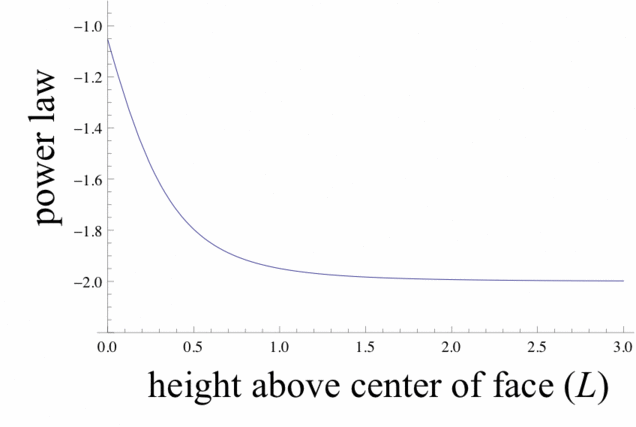At large distances (>1 or 2L), the force falls off as 1/r^2. At this distance, the particular shape doesn’t really matter. But at closer distance, the force falls off more slowly, approaching 1/r near the surface.

### What about equipotential surfaces, extent of oceans and atmospheres, and escape velocity?

I was unable to analytically find an expression for the gravitational potential of a cube, so I just had Mathematica numerically integrate the gravitational field along a path from the origin to the point of interest. From this, we can find quantitative values of the potential. Also, the equipotential lines can be evaluated numerically by solving a differential equation to find lines that are always perpendicular to the gravitational field.

The figure below shows two equipotential lines, where the vertical axis extend upwards from the center of a face, and the horizontal axis extends to the middle of an edge. This close to the surface, the equipotential surfaces are quite circular (see below), so the equipotential surface that contains this line can be approximated by revolving the line about the vertical axis. From this surface of revolution, we can calculate the volume contained between the cube and this surface. The purple line in the figure shows the approximate surface of the ocean, if the earth’s water were divided equally among the faces. If the atmosphere is such that pressure is 1 atm at the surface of the ocean, and we assume that pressure decreases exponentially (a pretty good approximation), then the boundary between “atmosphere” and “space” would be approximately at the blue equipotential line. (This boundary is chosen to be at the same pressure as at 100km above the spherical earth’s surface.) Interestingly, we see that the large majority of the cube’s surface is outside the atmosphere. Since humans can survive only at about 10km above the earth’s surface, this means that the habitable land on the cube would be a narrow ring around the oceans, about 10km wide.It is interesting to take a look at what the equipotential surfaces look like. The figure below shows 4 equipotential surfaces outside the cube at increasing values of the potential. The leftmost figure shows the shape of the oceans, and the second to left figure shows the boundary of the atmosphere, as described above.We can now easily calculate the radius of curvature of the equipotential surfaces at any point. The figure below shows the radius of curvature R of the equipotential surface at a point a distance x from the center of the cube, going towards the center of a face. For comparison, the radius of curvature in a spherically symmetric potential is shown, with the particular value of the earth’s radius indicated.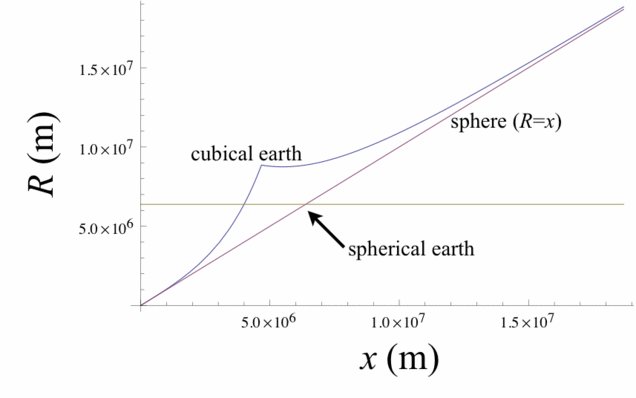We see that when the equipotential surface is far from the surface of the cube, the curvature is about the same as if the mass were spherically symmetric. Near the surface (where we see the kink), the radius of curvature increases, meaning that the equipotential surface is flattened out, reflecting the shape of the cube.

In particular, the radius of curvature at the top of the ocean is about 8830 km, (For the surface of the oceans, we can assume that the surface is spherical, so the radius of curvature is the same everywhere.) Note that the top of the ocean is about 4770km from the center of the cube, so this is quite near the kink above. This radius of curvature is larger than the radius of curvature of the spherical earth (6400km). This results in a horizon that would be slightly farther away, as seen from the same height above the water. (At 10m above the surface of the ocean, the horizon is 13km away on the cube and 11km away on earth.) An interesting observation is that if you were sailing in a ship from the center of the ocean towards the shore, the first land to come in to view would be a very distant edge or vertex of the cube. A vertex could come into view in as little as 130 km from the center of the ocean. The shore would not come into view until it was over the horizon, about 1300km from the center of the ocean (and 13km from shore).

With the gravitational potential, we can also easily calculate the escape velocity – the velocity you would need to give to an object somewhere on the cube so that it will move away from the cube forever (neither fall back down nor go into orbit). This is just the velocity where kinetic energy is equal to the change in potential energy from the surface to infinity. At a vertex, escape velocity is about 6050 m/s. At the center of a face, it is about 7470 m/s. This can be compared to escape velocity on the surface of the earth, about 11000 m/s. If the cube is rotating with a period of 24 hours, the velocity of any point on the cube will be much less than escape velocity (which also the case for spherical earth). In the best case scenario, if the cube is rotating about an axis through the middle of two opposite edges, then farthest out point at one of the vertices is moving at about 600m/s.

# Q: What would Earth be like to us if it were a cube instead of spherical? Is this even possible?

Physicist: The Earth is really round.  It’s not the roundest damn thing ever, but it’s up there.  If the Earth were the size of a basketball our mountains and valleys would be substantially smaller than the bumps on the surface of that basketball.  And there’s a good reason for that.

Rocks may seem solid, but on a planetary scale they’re squishier than soup.  A hundred mile column of stone is freaking heavy, and the unfortunate rocks at the bottom are going to break in a hurry.  Part of what keeps mountains short is erosion, but a bigger component is that the taller a mountain is the more it tends to sink under its own weight.  So as a planet gets bigger, and gets more gravity, the weight of the material begins to overwhelm the strength of that material, and the planet is pulled into a sphere.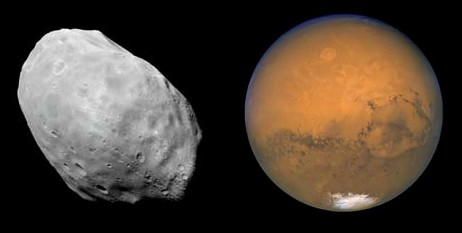Phobos (left), a very small moon, isn’t big enough to generate the gravity necessary to crush itself into a sphere. Unlike its host planet Mars (right).

So a tiny planet could be cube shaped (it’s not likely to form that way, but whatev’s).  Something the size of the Earth, however, is doomed to be hella round.

Life on a cubic Earth would be pretty different.  Although gravity on the surface wouldn’t generally point toward the exact center of the Earth anymore (that’ a symptom of being a sphere), it will still point roughly in toward the center.  So, the closer you are to an edge, the more gravity will make it feel as though you’re on a slope.  So, although it won’t look like it, it will feel like each of the six sides forms a bowl.  This has some very profound effects.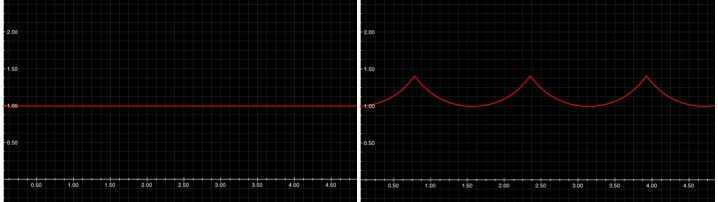If you walk around the Earth’s equator (left) your altitude says almost perfectly even. If you walk around the cube-Earth’s equator, cutting four of the faces in half, you’d experience “altitudes” as high as 2,600km (Everest is 8.8km). The 8 corners of the cube would be 4,700km higher than the centers of each face.

The seas and atmosphere would flow to the lowest point they can find and as such would puddle in a small region in the center of each face, no more than a thousand miles or so across.  However, both the seas and atmosphere would be several times deeper.  Which doesn’t count for as much as you might think.  Here on Earth (sphere-Earth), if you’re around 5km above sea level most of the air is below you.

The vast majority of the Earth would take the form of vast, barren expanses of rock, directly exposed to space.  If you were standing on the edge of a face, and looked back toward the center, you’d be able to clearly see the round bubble of air and water extending above the flat surface.  I strongly suspect that it would be pretty.

All life (land based life anyway) would be relegated to a thin ring around the shore of those bubble seas a couple dozen miles across.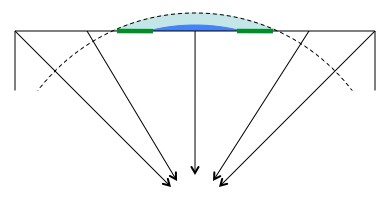Cross-section of a face: Gravity still points roughly toward the center of the cube-Earth. As a result the water (blue) and air (light blue) flows “downhill” and accumulates at the center of each face. The only land that could be inhabited is the land surounding the sea, where the air meets the ground (green lines). This picture is way out of scale. There is no where near this much air and water on our Earth.

Assuming that the cube was oriented in the way most people are probably imagining it right now, with the poles in the center of two of the faces, then two of those bubble seas would take the form of solid ice cap blocks.

What’s really cool is that the cube-Earth would have 6 completely isolated regions.  There’s no good reason, beyond some kind of “local panspermia“, for the life on each face to be related to the life on each of the other faces.  If the biospheres took different routes  you could even have a nitrogen/oxygen atmosphere on some faces (like we have) and a hydrogen/nitrogen/carbon-dioxide atmosphere on others (like our old atmosphere 3 billion years ago).

The small area would also affect (end) large-scale air and water movement.  You wouldn’t have to worry about hurricanes, but the cube-Earth would also have a really hard time equalizing temperature.  If you’ve jumped into the Pacific Ocean on the west coast (of the United States) you’re familiar with the teeth-chattering horror of the Arctic currents, and if you’ve been in the Atlantic Ocean on the east coast (USA again) you’re no doubt familiar with the surprisingly pleasant equatorial currents.  Point is: there’s a lot of thermal energy being carried around by the air and water.  On cube-Earth you’d have to deal with huge seasonal temperature fluctuations.

If I had to guess; it’s unlikely that complex life would evolve on a cube Earth.  However!  If it did, then their space program would be as easy as a long walk, and their handsomest physicists would spend their time pondering what a round Earth would be like.

By the by, the cube earth photo is by “Altered Realities“.

Update: The dude over at Possibly Wrong went through a bunch of the math behind the gravity of cube earths, and filled in a lot of the details that this post left out.  It’s clearly presented, and well worth a look: Possibly Wrong: If the Earth Were a Cube.

# If the Earth Were a Cube

What if the Earth were a cube instead of (approximately) a sphere?  I saw this same strange question twice in the last couple of weeks, first in a Reddit post linking to a goodAsk a Mathematician article, then again in a recent Straight Dope column, with the usual entertaining-while-informing reply from Cecil.  Both are very interesting reading, and I do not intend to rehash all of their observations here.  The purpose of this post is to provide some additional details that I found interesting, as well as to point out a couple of possible errors in the earlier write-ups.

I like “what if” questions like this, mostly because they are fun, but also because they are good exercise.  Thought experiments like this one often lead to additional or clearer insight into a more general problem.  In this case, what I found most interesting about this problem is how strange the effects of gravity would be on a cube-shaped planet; I learned that I did not understand gravity quite as well as I thought I did.  There is a reason why physicists prefer their chickens to be spherical.

But first, let’s define the problem.  For the most direct comparison with our experience, it seems reasonable to assume that our cube planet has the same mass and volume (and thus mean density) as the Earth, as in the figure below.

An immediately noticeable difference is the enormous range of altitudes.  Using the center of each face of the cube as a reference, each edge is approximately 1,300 miles (2,100 km) higher (i.e., farther from the center of the cube), and each corner is 2,300 miles (3,800 km) higher.  Compare this with the Earth, where altitudes vary over just tens of kilometers.

(The Ask a Mathematician article gives larger altitudes that suggest an assumption of a larger cube that contains the Earth, with a side length equal to the Earth’s diameter.  This would imply that the cube has either a larger mass or a smaller density than the Earth.)

Beyond just these geometric differences, the physical effects of gravity are even weirder.  First, of minimal weirdness is the observation that gravity is much weaker near the edges and corners than at the center of a cube face.  This makes sense, since the edges and corners are “farther away” from the center of mass of the cube.  The figure below shows the magnitude of the force of gravity over the surface of each cube face, normalized by 1 “Earth g“:

At the center of each cube face, the force of gravity is almost exactly 1 g; at each corner, however, it is just 0.646 g, meaning that a person weighing 200 lbs. here on Earth would weigh only 129 lbs.

(Using this same example, the Straight Dope article suggests that this weight is only 103 lbs.  This value assumes that the cube and the person at the corner are point masses, which is a safe assumption when the bodies in question are spherical.  But when they are not, things get complicated, even for relatively simple shapes like a cube, as we will see shortly.)

A slightly weirder effect is that, standing on the flat surface of a cube-shaped planet, the force of gravity is not always “down.”  That is, as you walk in a straight line from the center of a face toward a corner, gravity causes the flat face of the cube to seem to get steeper and steeper, so that you are eventually climbing instead of walking.  This also makes sense, since the force of gravity is directed approximately toward the center of the cube, which is only “straight down” at the center of each face:The “steepness” of the perceived hill, or the angle in degrees between the gravity vector and the cube surface normal.

Finally, I think the most interesting part of this problem, and what caught my attention in the first place, is the following innocuous statement in the Ask a Mathematician article:

“… Gravity on the surface wouldn’t generally point toward the exact center of the [cube] Earth anymore.”

In other words, when calculating the force of gravity exerted by the cube, even on a point mass, the direction of that force is not always toward the center of (mass of) the cube.  This was a surprise to me; I had to think about it for a while to realize that, even with all of the nice symmetry, constant density, etc., of the cube, the correspondingly “nice” Shell Theorem, or Gauss’ flux law, etc., do not help us here.  We essentially have to resort to the triple integral to work out exactly how gravity behaves on our cube-shaped planet.  The details of the derivation are at the end of this post.

And it is not a small effect.  I was surprised by just how much the direction of the force of gravity deviates from the center of the cube, nearly 14 degrees in some places, as shown in the figure below.  The overall effect is essentially to reduce the “steepness” effect described above, so that the force of gravity is directed more nearly straight down than directly toward the center of the cube.  As expected, the deviation is zero at the center of each face, at the center of each edge, and at the corners.The angle in degrees between the gravity vector and the vector to the center of the cube.

The Gravitational Potential for a Cuboid

I initially tried the brute-force numeric integration approach, but particularly for points near the surface of the cube where we are interested, the integrand is not very well-behaved.  At the other extreme, the Werner-Scheeres paper referenced below describes an interesting algorithm for computing the exact gravitational field for arbitrary polyhedra.  Fortunately, the cube is sufficiently simple that we can work it out by hand, with a little help from Mathematica.

We can generalize slightly by considering a cuboid with side lengths$(2a, 2b, 2c)$.  The acceleration due to gravity is the gradient of the potential function$U$ defined at a point$(x_0,y_0,z_0)$ by$U(x_0,y_0,z_0) = G\rho\int_{-a-x_0}^{a-x_0}\int_{-b-y_0}^{b-y_0}\int_{-c-z_0}^{c-z_0}\frac{1}{\sqrt{x^2+y^2+z^2}}dx\,dy\,dz$

where$G$ is the gravitational constant and$\rho$ is the density of the cube.  Note the change of variables to shift the origin to$(x_0,y_0,z_0)$, which has the convenient effect that the integrand does not involve any of the limits of integration.  So after each of the three integrations, we can eliminate any summation term that does not involve all threevariables x, y, and z, since the term will evaluate to zero in the final result.

Mathematica does most of the heavy lifting, with some nudging simplification, yielding the following expression for the potential function:$U(x_0,y_0,z_0) = G\rho(w(x,y,z)+w(y,z,x)+w(z,x,y))]_{x=-a-x_0}^{a-x_0}]_{y=-b-y_0}^{b-y_0}]_{z=-c-z_0}^{c-z_0}$$w(x,y,z) = x y \ln(z+\sqrt{x^2+y^2+z^2}) - \frac{1}{2}x^2 \arctan{\frac{y z}{x\sqrt{x^2+y^2+z^2}}}$

References:

1. R. Werner and D. Scheeres, Exterior Gravitation of a Polyhedron Derived and Compared With Harmonic and Mascon Gravitation Representations of Asteroid 4769 Castalia. Celestial Mechanics and Dynamical Astronomy, 65 (1997):313-44. [PDF]